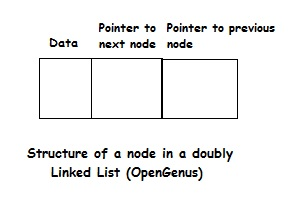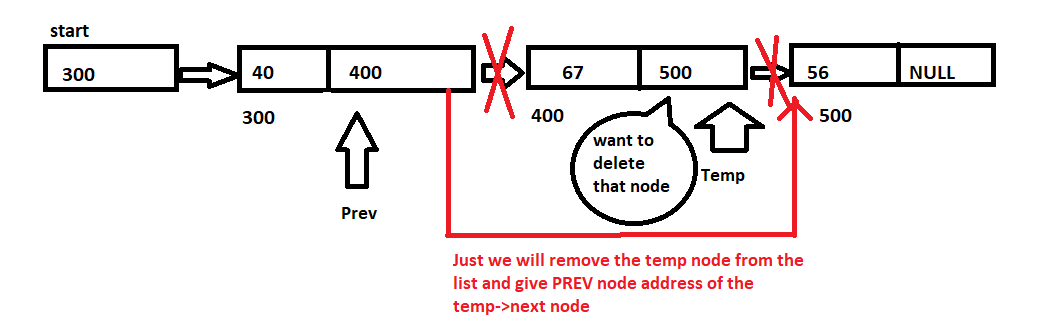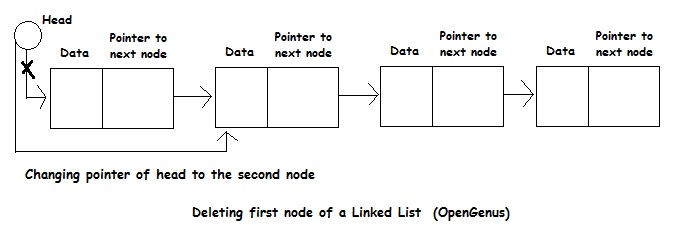×

Search anything:

# Deletion operation in a Linked ListReading time: 15 minutes | Coding time: 20 minutes

Linked List is an efficient data structure to store data. You can learn about the basics of Linked List data structure in this wonderful post. In this session, we will explore the deletion operation in a Linked List.

As we discussed previously, any data structure that stores data has three basic operations:

• Insertion: To insert data into the data structure
• Deletion: To delete data from the data structure
• Search: To search for a data in the data structure

In this leason, we will explore the deletion operation in a Linked List in depth.

The structure of a node in a Linked List is as follows:The structure of a node in a doubly Linked List is as follows:The variants of deletion operation that can be performed are:

• Deleting first node of the linked-list
• Deleting a node at before location given.
• Deleting a node after location is given.
• Deleting a last node of the linked list.

### How to delete a node?

To delete a node, we have to redirect the next pointer of the previous node to point to the next node instead of the current one. This is possible when we have access to the previous node.

If we do not have a pointer to the previous node, we cannot redirect its next pointer. In this case, we can copy the data of the next node to the current node and delete the next node.### How to delete first node of a Linked List?

The process of deleting a head of a Linked List is simple and same as the previous method of deleting any node of a Linked List.### How does remove tail from singly Linked List?

We need to iterate over the nodes of the list until node.next.next is null. At this point, node refers to the next to last node, and node.next refers to the last node. Setting node.next to null removes the last node from the list, since no node in the list refers to it anymore.

Given a pointer to a tail of a Linked List without any access to previous nodes makes it impossible to remove the tail from the Linked List.

### Pseudocode

Pseudocode of deleting a node from a doubly Linked List is as follows:

### Pseudocode to delete first node of a Linked List

``````
E unlinkFirst(Node<E> f) // Delete node f
{
final Node<E> next = f.next; // next points to the next node to f
first = next; // first is the pointer to the first node
final E element = f.item;
f.item = null;
f.next = null;
if(next == null) // Case of only one node
last = null;
else
next.prev = null;
-- size; // Reducing size of Linked List
return element;
}
``````

### Pseudocode to delete last node of a Linked List

``````
{
final E element = l.item;
final Node<E> newLast = l.prev;
l.prev = null;
l.item = null;
last = newLast;
if(newLast == null)
first = null;
else
newLast.next = null;
-- size;
return element;
}
``````

### Pseudocode to delete any node of a Linked List

``````
{
final E element = n.item;
final Node<E> before = n.prev;
final Node<E> next = n.next;
if( before == null )
else if(next == null)
else
{
n.item = null;
n.next = null;
n.prev = null;
before.next = next;
next.prev = before;
-- size;
}
return element;
}
``````

### Implementations

Implementation of deletion operation in a Linked List is as follows:

• Java

### Java

``````
import java.util.*;
{
private static class Node<E>
{
E item;
Node<E> next;
Node<E> prev;
Node(Node<E> prev, E element, Node<E> next)
{
this.item = element;
this.prev = prev;
this.next = next;
}
}
transient int size = 0;
transient Node<E> first;
transient Node<E> last;
// Creates an empty list
// Return Node at index "index" O(N) time
public Node<E> node( int index)
{
if(index < (size >> 1))
{
Node<E> x = first;
for(int i=0;i<index; ++i)
x = x.next;
return x;
}
else
{
Node<E> x = last;
for(int i=size-1; i>index; --i)
x = x.prev;
return x;
}
}
// Print all elements in the LinkedList
public void printList()
{
Node<E> x = first;
for(int i=0;i<size; ++i)
{
System.out.println(x.item);
x = x.next;
}
}
{
final Node<E> front = first;
final Node<E> newNode = new Node<>(null, e, front);
first = newNode;
if( front == null)
last = newNode;
else
front.prev = newNode;
++ size;
}
{
final Node<E> l = last;
final Node<E> newNode = new Node<>(l, e, null);
last = newNode;
if(l == null)
first = newNode;
else
l.next = newNode;
++ size;
}
// Inserts a node before a non null node succ
private void LinkBefore(E e, Node<E> succ)
{
Node<E> before = succ.prev;
Node<E> newNode = new Node<E>(before, e, succ);
succ.prev = newNode;
if(before == null)
{
first = newNode;
}
else
{
before.next = newNode;
}
++ size;
}
{
final Node<E> next = f.next;
first = next;
final E element = f.item;
f.item = null;
f.next = null;
if(next == null)
last = null;
else
next.prev = null;
-- size;
return element;
}
{
final E element = l.item;
final Node<E> newLast = l.prev;
l.prev = null;
l.item = null;
last = newLast;
if(newLast == null)
first = null;
else
newLast.next = null;
-- size;
return element;
}
{
final E element = n.item;
final Node<E> before = n.prev;
final Node<E> next = n.next;
if( before == null )
else if(next == null)
else
{
n.item = null;
n.next = null;
n.prev = null;
before.next = next;
next.prev = before;
-- size;
}
return element;
}
{
}
{
}
{
}
}
{
public static void main()
{
Node<Integer> nn = ll.node(3);
ll.printList();
}
}
``````

### Complexity

• Worst case time complexity: `Θ(1)`
• Average case time complexity: `Θ(1)`
• Best case time complexity: `Θ(1)`
• Space complexity: `Θ(1)`#### OpenGenus Tech Review Team

The official account of OpenGenus's Technical Review Team. This team review all technical articles and incorporates peer feedback. The team consist of experts in the leading domains of Computing.

Improved & Reviewed by:

Deletion operation in a Linked List